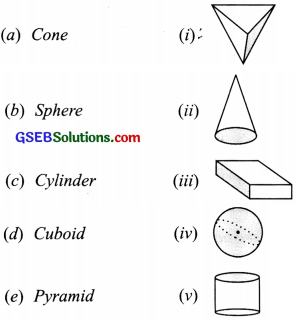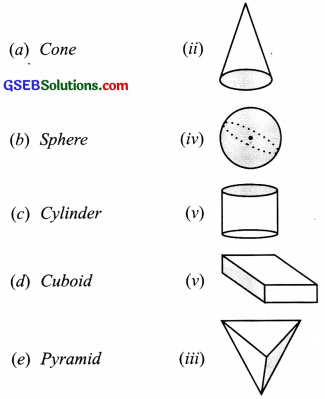# GSEB Solutions Class 6 Maths Chapter 5 Understanding Elementary Shapes Ex 5.9

Gujarat Board GSEB Textbook Solutions Class 6 Maths Chapter 5 Understanding Elementary Shapes Ex 5.9 Textbook Questions and Answers.

## Gujarat Board Textbook Solutions Class 6 Maths Chapter 5 Understanding Elementary Shapes Ex 5.9

Question 1.
Match the following:Give two new examples of each shape.
Solution:Other examples:
(a) Cone:
(i) An ice cream cone
(ii) A birthday cap

(b) Sphere:
(i) A cricket ball
(ii) A tennis ball

(c) Cylinder:
(i) A lawn-roller

(d) Cuboid:
(i) A brick
(ii) A book

(e) Pyramid:
(i) Pyramids of Egypt
(ii) A diamondQuestion 2.
What shape is: